# Matrix algebra

(diff) ← Older revision | Latest revision (diff) | Newer revision → (diff)

algebra of matrices

A subalgebra of the full matrix algebra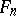of all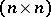-dimensional matrices over a field. The operations inare defined as follows: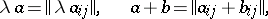where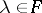, and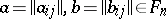. The algebrais isomorphic to the algebra of all endomorphisms of an-dimensional vector space over. The dimension ofoverequals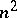. Every associative algebra with an identity (cf. Associative rings and algebras) and of dimension overat mostis isomorphic to some subalgebra of. An associative algebra without an identity and with dimension overless thancan also be isomorphically imbedded in. By Wedderburn's theorem, the algebrais simple, i.e. it has only trivial two-sided ideals. The centre of the algebraconsists of all scalar-dimensional matrices over. The group of invertible elements ofis the general linear group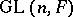. Every automorphismofis inner: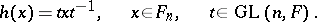Every irreducible matrix algebra (cf. also Irreducible matrix group) is simple. If a matrix algebrais absolutely reducible (for example, if the fieldis algebraically closed), then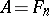for(Burnside's theorem). A matrix algebra is semi-simple if and only if it is completely reducible (cf. also Completely-reducible matrix group).

Up to conjugation,contains a unique maximal nilpotent subalgebra — the algebra of all upper-triangular matrices with zero diagonal entries. Inthere is an-dimensional commutative subalgebra if and only if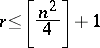(Schur's theorem). Over the complex fieldthe set of conjugacy classes of maximal commutative subalgebras of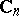is finite forand infinite for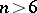.

Inone has the standard identity of degree: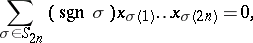wheredenotes the symmetric group and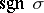the sign of the permutation, but no identity of lower degree.

How to Cite This Entry:
Matrix algebra. Encyclopedia of Mathematics. URL: http://encyclopediaofmath.org/index.php?title=Matrix_algebra&oldid=13522
This article was adapted from an original article by D.A. Suprunenko (originator), which appeared in Encyclopedia of Mathematics - ISBN 1402006098. See original article